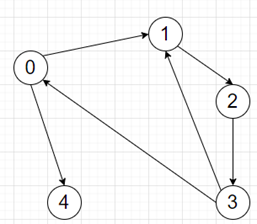# Program to find total cost for completing all shipments in python

Suppose we have a list of lists called ports, where ports[i] represents the list of ports that port i is connected to. We also have another list of lists called shipments where each list of the sequence [i, j] which denotes there is a shipment request from port i to port j. And the cost to ship from port i to port j is the length of the shortest path from the two ports, we have to find the total cost necessary to complete all the shipments.

So, if the input is like ports = [[1, 4],,,[0, 1],[]] shipments = [[1, 4]], then the output will be 4, as the path is from 1 -> 2 -> 3 -> 0 -> 4.To solve this, we will follow these steps -

• n := size of ports
• dist := adjacency matrix from the ports list
• for j in range 0 to n, do
• for i in range 0 to n, do
• for k in range 0 to n, do
• dist[i, k] = minimum of dist[i, k], dist[i, j] + dist[j, k]
• for all shipments in form [i, j] make a list dist[i,j] when dist[i,j] is not infinity
• return the sum of generated list.

Let us see the following implementation to get better understanding:

## Example

Live Demo

class Solution:
def solve(self, ports, shipments):
n = len(ports)
INF = 10 ** 10
dist = [[INF for _ in range(n)] for _ in range(n)]
for i in range(n):
dist[i][i] = 0
for i in range(n):
for j in ports[i]:
dist[i][j] = 1
for j in range(n):
for i in range(n):
for k in range(n):
dist[i][k] = min(dist[i][k], dist[i][j] + dist[j][k])

return sum(dist[i][j] for i, j in shipments if dist[i][j] != INF)

ob = Solution()
ports = [[1, 4],,,[0, 1],[]]
shipments = [[1, 4]]
print(ob.solve(ports, shipments))

## Input

[[1, 4],,,[0, 1],[]], [[1, 4]]

## Output

4